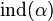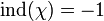# Frobenius-Schur indicator

Jump to: navigation, search

## Definition

Let$G$ be a finite group and$\alpha$ a character or virtual character of$G$. The Frobenius-Schur indicator of$\alpha$ is the value:$\operatorname{ind}(\alpha) = \sum_{x \in G} \alpha(x^2)/|G|$

Equivalently:$\operatorname{ind}(\alpha) = \sum \alpha(x^2)/|C_G(x)|$

where$x$ varies over a collection of conjugacy class representatives and$C_G(x)$ denotes the centralizer of$x$ in$G$.

Equivalently,$\operatorname{ind}(\alpha)$ is the inner product of$\alpha$ and the indicator character.

## Particular cases

### Examples where the Frobenius-Schur indicator is -1

Representation Computation of Frobenius-Schur indicator (section) Group Information on linear representation theory
faithful irreducible representation of quaternion group faithful irreducible representation of quaternion group#Frobenius-Schur indicator quaternion group linear representation theory of quaternion group
quaternionic representation of special linear group:SL(2,3) quaternionic representation of special linear group:SL(2,3)#Frobenius-Schur indicator special linear group:SL(2,3) linear representation theory of special linear group:SL(2,3)

## Facts

### For irreducible characters

Further information: Indicator theorem

If$\chi$ is an the character of an irreducible representation, then$\operatorname{ind}(\chi)$ is either 0, +1, or -1:

•$\operatorname{ind}(\chi) = +1$ if and only if$\chi$ is the character of a representation over$\R$
•$\operatorname{ind}(\chi) = -1$ if and only if$\chi$ is a real-valued character, but cannot be realized as the character of a real representation
•$\operatorname{ind}(\chi) = 0$ if and only if some value of$\chi$ is non-real